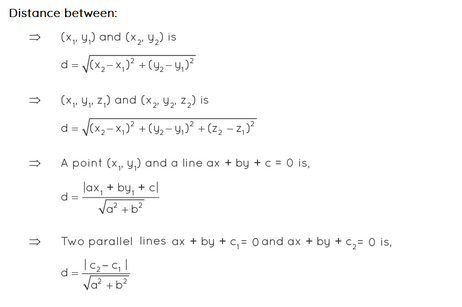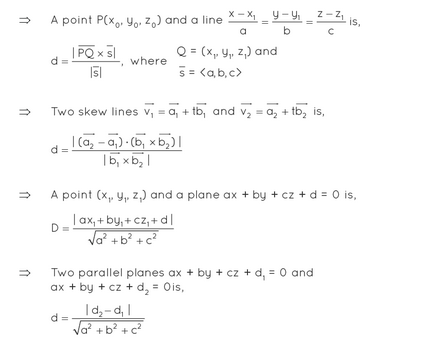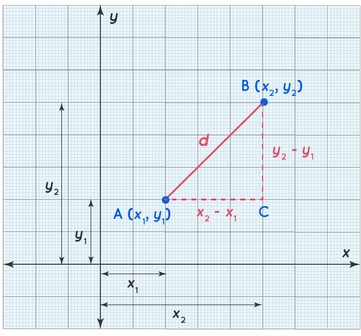Distance Formula homework Help at TutorEye

# Best Homework Help For Distance Formula

## Top Questions

2 test and it’s timed so i need it done in half an hour
View More

th and breadth of the field.
View More

ber being one out of three randomly picked lucky-numbers out of 75 before starting the round. 15 players, numbers 1-75, ticket containing 24 random numbers (5x5 with the middle filled).
View More

## Distance Formula:

The length of the line connecting two places is the distance between them.And the formula which is used to derive its length is called distance formula.

If the two locations are on the same horizontal or vertical line, the distance between them can be calculated by subtracting the non-overlapping coordinates.

## Distance Formula Frequently Asked Questions:

Question 1: What is the distance formula?

The length of the line segment connecting two points is equal to their distance.The Pythagoras theorem is used to obtain the formula for the distance between two points in a two-dimensional plane, which may also be used to estimate the distance between two points in a three-dimensional plane.

Question 2: What is the distance formula?

In coordinate geometry, there are several different forms of distance formulas which are listed below:Question 3: What is the distance formula?

Let us assume the points A(x1, y1) and B(x2, y2)

Let ‘d’ be the distance between A and B.

By using pythagoras theorem

AB2 = AC2 + BC2

d2 = (x- x1) + (y-  y1)

d = √(x- x1) + (y-  y1)Question 4: What is the distance formula?

A(5,2) and B(8,11)

AB2 = (8-5)2 + (11-2)2

AB = √(3)2 + (9)2 = √9 + 81 = 3√10